# Additive composition of natural numbers and its interesting properties

## Introduction

Any natural number can be expressed in terms of a unique set of prime numbers, the multiplication of which gives the original number. For prime numbers, this set consists of one element – the number itself. Such a composition can be called multiplicativeit is very well known and studied.

The article proposes a way to express a natural number in terms of a unique set of prime numbers (including one), the addition of which gives the original number. Such a composition will be called additiveand has not been previously offered.

When working on the article, the composition of numbers up to one trillion was calculated. This calculation gave quite interesting results, presented in the article. Perhaps a discussion of these results will help draw further conclusions suitable for publication in a scientific journal.

Let us introduce the concept of a primitive number. The natural number will be called primitiveif it is prime or equal to one. We will decompose a natural number into primitive ones according to the following rule:

Let be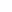– natural number. Find the largest primitive number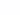such that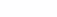. Compute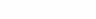. Then, similarly, we find the largest primitive number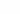such that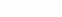. We will repeat these steps until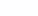will not be equal to zero; then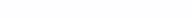A bunch of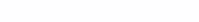will be called the additive composition of the number.

An additive partition, like a multiplicative partition, is single-valued for each. The breakdown for the first 50 numbers looks like this:

 1 = 1*2 = 1+13 = 2+14 = 3+15 = 3+26 = 5+17 = 5+28 = 7+19 = 7+210 = 7+3 11 = 7+3+112 = 11+113 = 11+214 = 13+115 = 13+216 = 13+317 = 13+3+118 = 17+119 = 17+220 = 19+1 21 = 19+222 = 19+323 = 19+3+124 = 23+125 = 23+226 = 23+327 = 23+3+128 = 23+529 = 23+5+130 = 29+1 31 = 29+232 = 31+133 = 31+234 = 31+335 = 31+3+136 = 31+537 = 31+5+138 = 37+139 = 37+240 = 37+3 41 = 37+3+142 = 41+143 = 41+244 = 43+145 = 43+246 = 43+347 = 43+3+148 = 47+149 = 47+250 = 47+3

* this partition is an exception, it is not considered strictly according to the formula

The only case when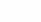there are identical numbers, is 2 = 1 + 1, then the numbers do not repeat. This is presumably due to the fact that betweenand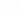there is at least one prime number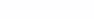.

As you can see, the sizefor the first numbers (except one) is two or three. The first composition of length 4 appears at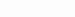(1354 = 1327+23+3+1), the first prime number with composition of length 4 is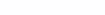(2010881 = 2010733+139+7+2).

And that is all! All numbers up to one trillion inclusive are divided into no more than 4 numbers. The first of the immediately arising questions is whether all natural numbers in general can be divided into no more than 4 primitive numbers?

Suppose there are two prime numbers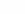andsuch that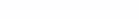. Then the partitionwill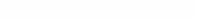. Apparently, there are no “forbidden” distances between primes, and sooner or later there will be a distance of 1354 and the second number will have a composition of length 5. How far is the first such pair? Wikipedia He speaksthat the distance 1356 is somewhere in the region of four hundred quadrillion.

Humor: does anyone have a supercomputer in mind to run the program to such a value? And where in general to write down the information on such amount of prime numbers? To write numbers up to a trillion, a bitmap of 116 gigabytes in size was used.

The second interesting property is that for primes up to a trillion, all compositions of length 4 end in 7+2. Depending on the distance between the primes, the last three numbers look like this (distance = x+7+2):

 122 = 113+7+2148 = 139+7+2190 = 181+7+2208 = 199+7+2220 = 211+7+2250 = 241+7+2292 = 283+7+2 302 = 293+7+2326 = 317+7+2346 = 337+7+2418 = 409+7+2430 = 421+7+2476 = 467+7+2532 = 523+7+2

A second similar question is: is this true for all primes with composition of length 4?

## Conclusion

The idea of ​​additive composition was born in the process of thinking about the topic: is it possible to understand what the next prime number is if all the prime numbers before it are known? Those. calculate the next prime number using a simple formula or algorithm. When the additive composition was formulated, the natural desire was to calculate it in practice and look at the results.

The purpose of the article is to involve other interested participants in the study.